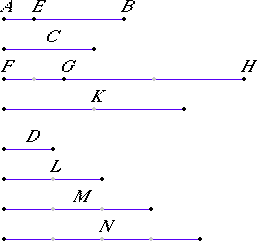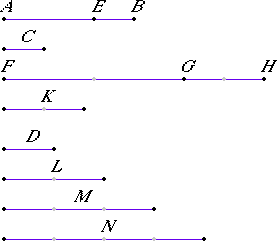# Proposition 8

Of unequal magnitudes, the greater has to the same a greater ratio than the less has; and the same has to the less a greater ratio than it has to the greater.

Let AB and C be unequal magnitudes, and let AB be greater, and let D be another, arbitrary, magnitude.

I say that AB has to D a greater ratio than C has to D, and D has to C a greater ratio than it has to AB.(V.Def.4)

Since AB is greater than C, make EB equal to C. Then the less of the magnitudes AE and EB, if multiplied, will eventually be greater than D.

First, let AE be less than EB. Let AE be multiplied, and let FG be a multiple of it which is greater than D. Make GH the same multiple of EB and K the same multiple of C that FG is of AE.

(V.Def.4)

Take L double of D and M triple of it, and successive multiples increasing by one, until what is taken is the first multiple of D that is greater than K. Let it be taken, and let it be N which is quadruple of D and the first multiple of it greater than K.

Since K is less than N first, therefore K is not less than M.

V.1

And, since FG is the same multiple of AE that GH is of EB, therefore FG is the same multiple of AE that FH is of AB.

But FG is the same multiple of AE that K is of C, therefore FH is the same multiple of AB that K is of C. Therefore FH and K are equimultiples of AB and C.

Again, since GH is the same multiple of EB that K is of C, and EB equals C, therefore GH equals K.

But K is not less than M, therefore neither is GH less than M.

And FG is greater than D, therefore the whole FH is greater than the sum of D and M.

But the sum of D and M equals N, inasmuch as M is triple D, and the sum of M and D is quadruple D, while N is also quadruple D, therefore the sum of M and D equals N.

But FH is greater than the sum of M and D, therefore FH is in excess of N, while K is not in excess of N.

V.Def.7

And FH and K are equimultiples of AB and C, while N is another, arbitrary, multiple of D, therefore AB has to D a greater ratio than C has to D.

I say next, that D has to C a greater ratio than D has to AB.

With the same construction, we can prove similarly that N is in excess of K, while N is not in excess of FH.

V.Def.7

And N is a multiple of D, while FH and K are other, arbitrary, equimultiples of AB and C, therefore D has to C a greater ratio than D has to AB.Next, let AE be greater than EB.

(V.Def.4)

Then the less, EB, if multiplied, will eventually be greater than D.

Let it be multiplied, and let GH be a multiple of EB and greater than D. Make FG the same multiple of AE, and K the same multiple of C that GH is of EB.

(V.Def.4)

Then we can prove similarly that FH and K are equimultiples of AB and C, and, similarly, take N the first multiple of D that is greater than FG, so that FG is again not less than M.

But GH is greater than D, therefore the whole FH is in excess of the sum of D and M, that is, of N.

Now K is not in excess of N, inasmuch as FG also, which is greater than GH, that is, than K, is not in excess of N.

And in the same manner, by following the above argument, we complete the demonstration.

Therefore, of unequal magnitudes, the greater has to the same a greater ratio than the less has; and the same has to the less a greater ratio than it has to the greater.

Q.E.D.

## Guide

Although the statement of this proposition is easy to comprehend, its proof is difficult. It says that if x > y, then x : z > y : z but z : x < z : y. Its converse is proposition V.10.

At four points in the proof V.Def.4 is used as an axiom of comparability rather than a definition. The first instance:

Then the less of the magnitudes AE and EB, if multiplied, will eventually be greater than D.
In fact the axiom of comparability is required for this proposition since it is false when infinitesimals are allowed. When y is infinitesimal with respect to x, then the first statement of the proposition doesn’t hold since x > x – y but it is not the case that x : x > (x – y):x, and the second statement doesn’t hold since x + y > x, but not x : x > x:(x + y).

#### Explanation of the proof

The proof is slightly more comprehensible when modern algebraic notation is used since that clarifies its overall structure. Every magnitude in Euclid’s proof is represented by a name and illustrated by a line. With an algebraic notation, we can refer to a magnitude by a formula. For instance, if we let a be AB and c be C, then we can use a – c for AE, thus reducing the number of variables and easing comprehension. We can also have variables for numbers, instead of having to choose a specific number as Euclid does when he takes N to be 4D.

But algebra obscures much, too. Euclid carefully proved distributivity of multiplication by numbers over addition of magnitudes in V.1, which is used in this proof. We manipulate algebraic expressions almost automatically. In order to be as correct as Euclid, we should verify the rules of algebra and be aware when we use them.

With these preliminary qualifications, let’s look at a translation of the proof into symbolic algebra.
 To prove: if a > c, then a : d > c : d, but d : c > d : a. a = AB c = C = EB d = D Let a > c. Either a – c < c, or a – c  > c, [or a – c = c]. a – c = AE Case 1: Suppose a – c < c. Let m be a number such that m(a – c) > d. m(a – c) = FG mc = GH = K Let n be the smallest number such that nd > mc. [What happens when n = 1 to Euclid’s proof?] nd = N (n – 1)d = M Since mc is not less than (n – 1)d, and m(a – c) > d, therefore, by adding, ma > nd. But mc is not greater than nd. Therefore a : d > c : d. ma = FH Also nd > mc but nd is not greater than ma. Therefore d : c > d : a. Case 2: Suppose c < a – c. Let Let m be a number such that mc > d. (Same as above) Let n be the smallest number such that nd > m(a – c). Since m(a – c) is not less than (n – 1)d, and mc > d, therefore, by adding, ma >  nd. [Euclid says to do the rest in the same manner: Since a – c  > c, therefore m(a – c)  > mc. But nd > m(a – c), therefore nd > mc. But ma >  nd, therefore a : d > c : d. Also nd > mc but nd is not greater than ma. Therefore d : c > d : a.] [Case 3 when a – c = c is left to the reader.] Thus, the conclusion is reached in any case. Q.E.D.

#### Use of this proposition

Proposition V.8 is used a few times in Book V starting with the next proposition.